# Algebra 1 : How to divide monomial quotients

## Example Questions

### Example Question #65 : Monomials

Divide the following monomial quotients: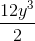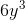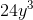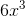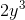Explanation:

To solve this problem, split it into two steps:

1. Divide the coefficients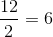2. Combine this with the lone variable to get the final answer:### Example Question #66 : Monomials

Divide the folloiwng monomial quotients: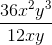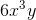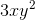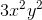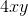Explanation:

To solve this problem, split it into two steps:

1. Divide the coefficients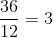2. Divide the variables. We also need to remember the following laws of exponents rule: When dividing variables, subtract the exponents.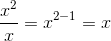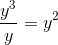Combine these to get the final answer:### Example Question #67 : Monomials

Divide the following monomial quotients: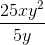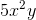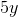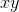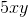Explanation:

To solve this problem, split it into two steps:

1. Divide the coefficients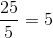2. Divide the variables. We also need to remember the following laws of exponents rule: When dividing variables, subtract the exponents.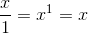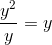Combine these to get the final answer:### Example Question #68 : Monomials

Divide the following monomial quotients: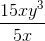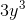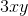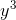Explanation:

To solve this problem, split it into two steps:

1. Divide the coefficients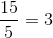2. Divide the variables. We also need to remember the following laws of exponents rule: When dividing variables, subtract the exponents.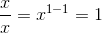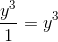Combine these to get the final answer:### Example Question #69 : Monomials

Divide the following monomial quotients: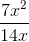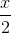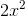Explanation:

To solve this problem, split it into two steps:

1. Divide the coefficients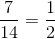2. Divide the variables. We also need to remember the following laws of exponents rule: When dividing variables, subtract the exponents.Combine these to get the final answer:### Example Question #70 : Monomials

Divide the following monomial quotients: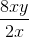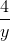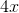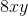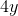Explanation:

To solve this problem, split it into two steps:

1. Divide the coefficients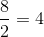2. Divide the variables. We also need to remember the following laws of exponents rule: When dividing variables, subtract the exponents.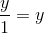Combine these to get the final answer:### Example Question #71 : Monomials

Divide the following monomial quotients: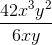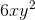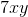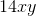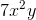Explanation:

To solve this problem, split it into two steps:

1. Divide the coefficients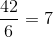2. Divide the variables. We also need to remember the following laws of exponents rule: When dividing variables, subtract the exponents.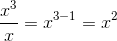Combine these to get the final answer:### Example Question #72 : Monomials

Divide the following monomial quotients: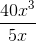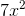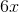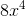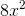Explanation:

To solve this problem, split it into two steps:

1. Divide the coefficients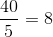2. Divide the variables. We also need to remember the following laws of exponents rule: When dividing variables, subtract the exponents.Combine these to get the final answer:### Example Question #73 : Monomials

Divide the following monomial quotients: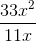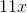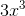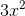Explanation:

To solve this problem, split it into two steps:

1. Divide the coefficients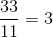2. Divide the variables. We also need to remember the following laws of exponents rule: When dividing variables, subtract the exponents.Combine these to get the final answer:### Example Question #74 : Monomials

Divide the following monomial quotients: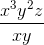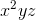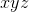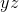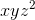Explanation:

To solve this problem, divide the variables. We also need to remember the following laws of exponents rule: When dividing variables, subtract the exponents.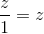Combine these to get the final answer: